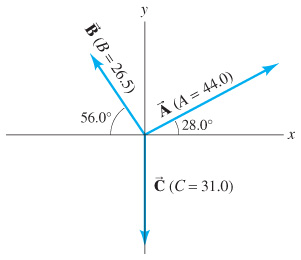Physics Practice Problems Vector Addition & Subtraction Practice Problems Solution: Three vectors are shown in Fig. 3-38. Their magnit...

🤓 Based on our data, we think this question is relevant for Professor Catala's class at MDC.

# Solution: Three vectors are shown in Fig. 3-38. Their magnitudes are given in arbitrary units. Determine the sum of the three vectors. Give the resultant in terms of:(a) components(b) magnitude and angle with x-axis.

###### Problem

Three vectors are shown in Fig. 3-38. Their magnitudes are given in arbitrary units. Determine the sum of the three vectors. Give the resultant in terms of:
(a) components
(b) magnitude and angle with x-axis.#### Q. A 650-N force acts in a northwesterly direction.A second 650-N force must be exerted in what direction so that the resultant of the two forces points ...

Solved • Mon Oct 29 2018 19:00:32 GMT-0400 (EDT)

#### Q. A plumber steps out of his truck, walks 66 m east and 35 m south, and then takes an elevator 12 m into the subbasement of a building where a bad leak ...

Solved • Mon Oct 29 2018 19:00:25 GMT-0400 (EDT)